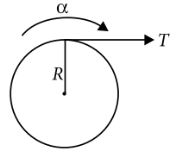Dynamics of rotational motion about fixed axis
Question

# A solid cylinder of mass 50 kg and radius 0.5 m is free to rotate about the horizontal axis. A massless string is wound round the cylinder with one end attached to it and other hanging freely. Tension in the string required to produce an angular acceleration of 2 revolutions  ${s}^{-2}$is

Moderate
Solution

##Here, mass of the cylinder, M = 50 kg Radius of the cylinder, R = 0.5 mMoment of inertia of the solid cylinder about its axis,$I=\frac{1}{2}M{R}^{2}$$\alpha =\frac{\tau }{I}=\frac{TR}{\frac{1}{2}M{R}^{2}}$$T=\frac{MR\alpha }{2}=\frac{50×0.5×4\pi }{2}=157\mathrm{N}$

Get Instant Solutions